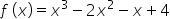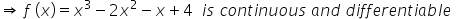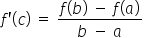Maths-
General
Easy

Question

# The value of 'c' in Lagrange's mean value theorem forin [0, 1] is

## 1/3    1/2    2/3    0Hint:

## The correct answer is: 1/3

### Given :is a polynomial in the range [0, 1]We know that a polynomial in the range is continuous and differentiableBy Lagrange's Mean Value Theorem Lagrange mean value theorem states that for any two points on the curve there exists a point on the curve such that the tangent drawn at this point is parallel to the secant through the two points on the curve.#### With Turito Foundation.#### Get an Expert Advice From Turito.